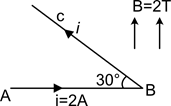A B C is a current carrying conductor carrying a current i = 2A. If length of the segment AB is 33m, then what should be the length of BC, so that net force on the conductor ABC becomes zero?

# A B C is a current carrying conductor carrying a current i = 2A. If length of the segment AB is $3\sqrt{3}$m, then what should be the length of BC, so that net force on the conductor ABC becomes zero?1. A

2. B

3. C

4. D

Register to Get Free Mock Test and Study Material

+91

Verify OTP Code (required)

### Solution:

Where le = Ac = equivalent length. It is evident that $\stackrel{\to }{\mathrm{F}}$ is zero when $\stackrel{\to }{{l}_{e}}$ and $\stackrel{\to }{\mathrm{B}}$ are parallel (as shown in the figure).Register to Get Free Mock Test and Study Material

+91

Verify OTP Code (required)